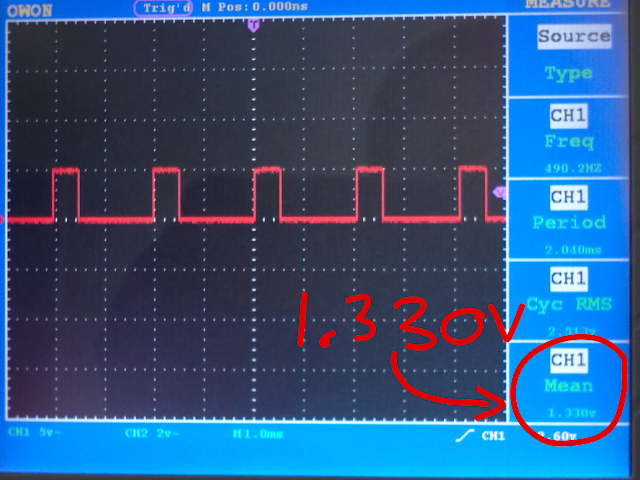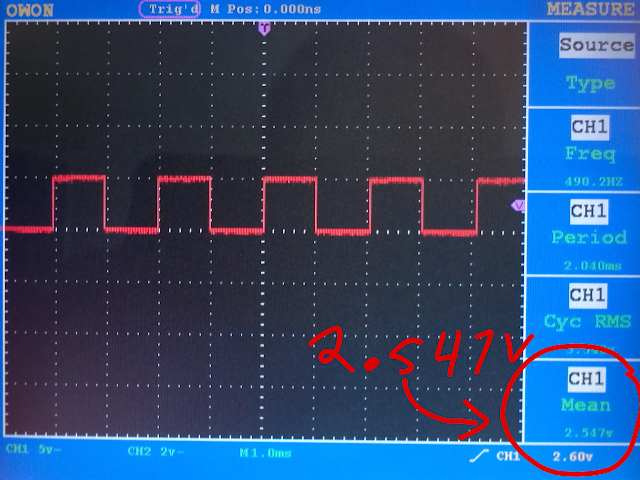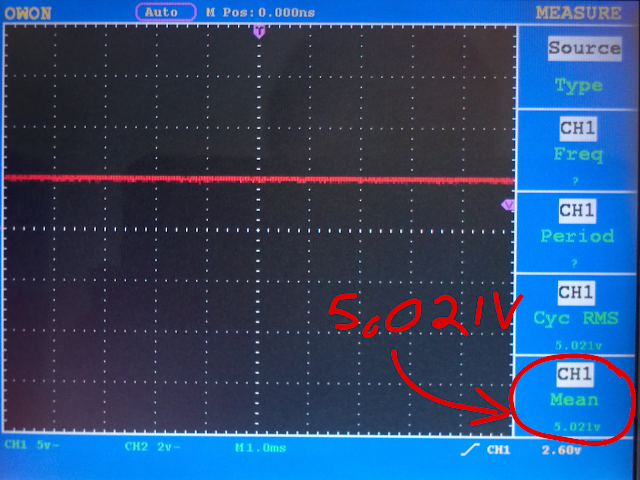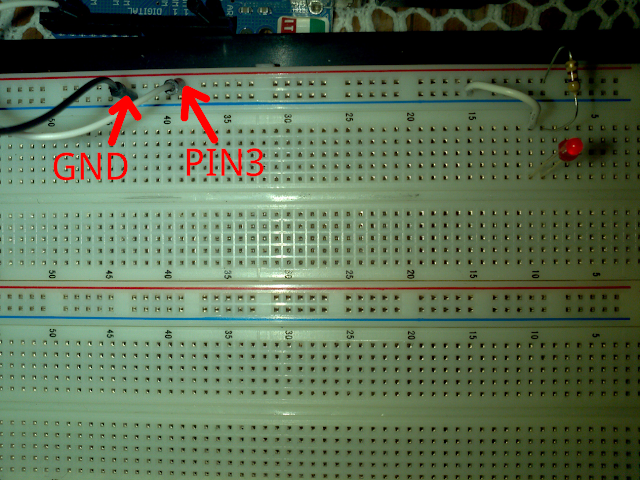# Control the Brightness of An LED With PWM From Arduino

by on May 9, 2011

in Arduino

You can turn on an LED, make it blink at any speed, it’s time you learn how to control it’s brightness using pulse width modulation.

## PWM: Pulse Width Modulation:

KISS, This just means changing the width of a square wave.

## The analogWrite() Function:

The Arduino uses 8 bits to represent analog data, this means that it can represent this data in decimal notation using any number between zero and 2^8-1 that is from 0 to 255, where 0 is zero volts and 255 is 5 volts. Any other number between 0 and 255 will represent its respective voltage between 0V and 5V.

To write analog data to a pin in the Arduino we use the command analogWrite(pin, value); where pin the is the pin you want to use for analog data, you can only use the pins marked &lquo;PWM&rquo;, and value is any number between 0 and 255. Throughout this tutorial I will be using pin 3.

## The Big Picture

I used an oscilloscope to take the screenshots, but you won’t need one.

Let’s see what happens when we write analogWrite(3, 255/4);A PWM wave

What we get is a square wave on pin 3 whose width was divided by 4, the full width will produce 5 volts so this wave will produce 1.25 volts (ideally, nothing is perfect in really life as you can see we actually got 1.3 volts)

Here are more examples so you can grasp the idea better.PWM wave producing 2.5 volts

We got the wave above by doing analogWrite(3, 255/2); . This one is pretty close to its theoretical value (2.5V)

Now let’s try analogWrite(analogPin, 255); to get the full 5V. Here is the picture.PWM wave at full width

## Bring On the LED!

Now, to control the brightness of an led, which is the whole point of this tutorial, all you have to do is hook up your favorite led to pin3 through a resistor and to ground.led brightness control circuit

and of course here is a snippet of code which will dim the led in and out.

```int analogPin=3;
int i=0;

void setup()
{

}

void loop()
{
for(i=0;i<255;i++)
{
analogWrite(analogPin, i);
delay(5);
}

for( ; i>0;i--)
{
analogWrite(analogPin, i);
delay(5);
}

}
```

## The Result:

Of course am no charlatan, here is proof that this actually works.

Notice how the LED’s brightness is proportional to the width of the wave.